# Microteaching PPT.pptx

Mar. 28, 2023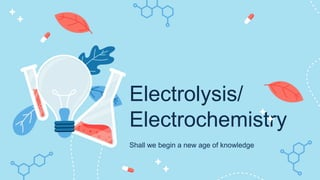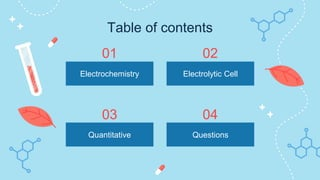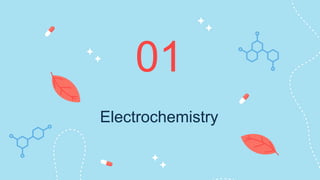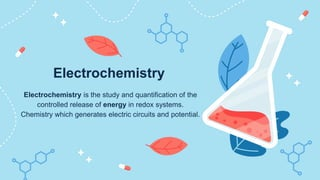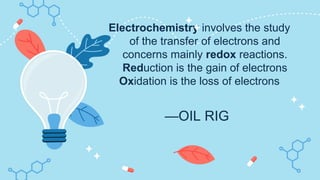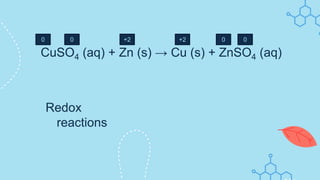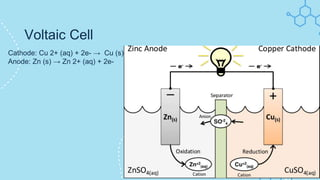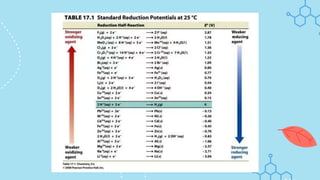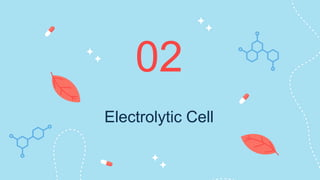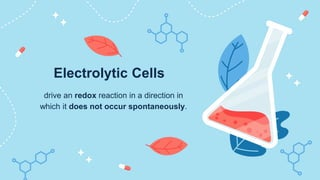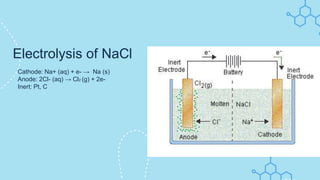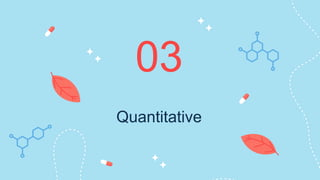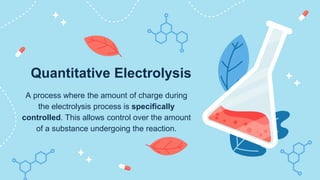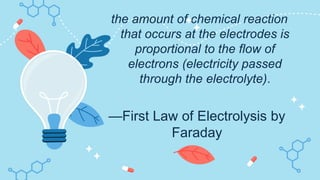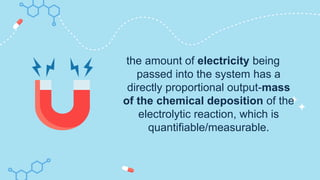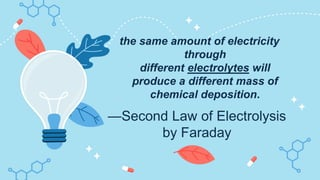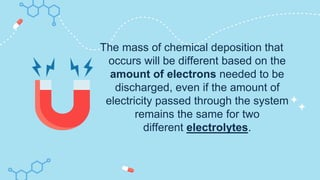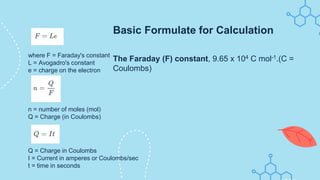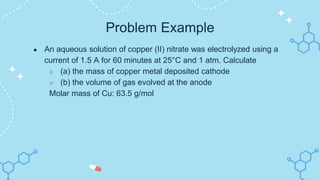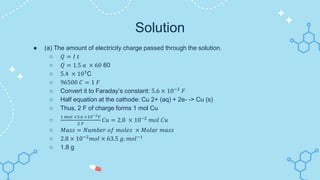1 of 21

### Microteaching PPT.pptx

1. Electrolysis/ Electrochemistry Shall we begin a new age of knowledge
3. Electrochemistry 01
4. Electrochemistry is the study and quantification of the controlled release of energy in redox systems. Chemistry which generates electric circuits and potential. Electrochemistry
5. —OIL RIG Electrochemistry involves the study of the transfer of electrons and concerns mainly redox reactions. Reduction is the gain of electrons Oxidation is the loss of electrons
6. Redox reactions CuSO4 (aq) + Zn (s) → Cu (s) + ZnSO4 (aq) 0 +2 0 +2 0 0
7. Cathode: Cu 2+ (aq) + 2e- → Cu (s) Anode: Zn (s) → Zn 2+ (aq) + 2e- Voltaic Cell
8. Electrolytic Cell 02
9. drive an redox reaction in a direction in which it does not occur spontaneously. Electrolytic Cells
10. Cathode: Na+ (aq) + e- → Na (s) Anode: 2Cl- (aq) → Cl2 (g) + 2e- Inert: Pt, C Electrolysis of NaCl
11. Quantitative 03
12. A process where the amount of charge during the electrolysis process is specifically controlled. This allows control over the amount of a substance undergoing the reaction. Quantitative Electrolysis
13. —First Law of Electrolysis by Faraday the amount of chemical reaction that occurs at the electrodes is proportional to the flow of electrons (electricity passed through the electrolyte).
14. the amount of electricity being passed into the system has a directly proportional output-mass of the chemical deposition of the electrolytic reaction, which is quantifiable/measurable.
15. —Second Law of Electrolysis by Faraday the same amount of electricity through different electrolytes will produce a different mass of chemical deposition.
16. The mass of chemical deposition that occurs will be different based on the amount of electrons needed to be discharged, even if the amount of electricity passed through the system remains the same for two different electrolytes.
17. where F = Faraday's constant L = Avogadro's constant e = charge on the electron n = number of moles (mol) Q = Charge (in Coulombs) The Faraday (F) constant, 9.65 x 104 C mol-1.(C = Coulombs) Q = Charge in Coulombs I = Current in amperes or Coulombs/sec t = time in seconds Basic Formulate for Calculation
18. Problem Example ● An aqueous solution of copper (II) nitrate was electrolyzed using a current of 1.5 A for 60 minutes at 25°C and 1 atm. Calculate ○ (a) the mass of copper metal deposited cathode ○ (b) the volume of gas evolved at the anode Molar mass of Cu: 63.5 g/mol
19. Solution ● (a) The amount of electricity charge passed through the solution. ○ 𝑄 = 𝐼 𝑡 ○ 𝑄 = 1.5 𝑎 × 60 60 ○ 5.4 × 103 C ○ 96500 𝐶 = 1 𝐹 ○ Convert it to Faraday’s constant: 5.6 × 10−2 𝐹 ○ Half equation at the cathode: Cu 2+ (aq) + 2e- -> Cu (s) ○ Thus, 2 F of charge forms 1 mol Cu ○ 1 𝑚𝑜𝑙 ×5.6 ×10−2𝐹 2 𝐹 𝐶𝑢 = 2.8 × 10−2 𝑚𝑜𝑙 𝐶𝑢 ○ 𝑀𝑎𝑠𝑠 = 𝑁𝑢𝑚𝑏𝑒𝑟 𝑜𝑓 𝑚𝑜𝑙𝑒𝑠 × 𝑀𝑜𝑙𝑎𝑟 𝑚𝑎𝑠𝑠 ○ 2.8 × 10−2 𝑚𝑜𝑙 × 63.5 𝑔. 𝑚𝑜𝑙−1 ○ 1.8 g
20. Question 04